# a) find the no-load value of v0 in the circuit shown b) find v0 when RL...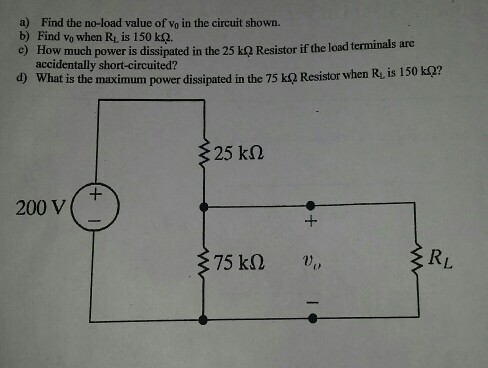a) find the no-load value of v0 in the circuit shown
b) find v0 when RL is 150 k(ohms)
c) how much power is dissipated in the 25 k(ohms) resistor if the load terminals are accidentally short-circuited?
d) what is the maximum power dissipated in thr 75 k(ohms) resistor when RL is 150 k(ohms)?

a) Find the no-load value of vo in the circuit shown. b) Find vo when Rt is 150 kQ. c) How much power is dissipated in the 25㏀ Resisto r if the load terminals are accidentally short-circuited? d) What is the maximun power dissipated in the 75 ㏀ Resistor when R, is 150 ㏀? 25 kΩ 200 V RL

A) no load value of Vo =200*75/(75+25) =150V

B)when RL =150kOhm

Rparallel = 75*150/(75+150)

= 50 kohm

Vo = 200*50/(50+25)

= 133.3 V

C) if short circuited, only resistance in the circuit will be 25kohm

Power = V^2/R = 200^2/25000 = 1.6 W

D) Maximum power = V^2/R = 133.3^2/75000

= 0.237 W

##### Add Answer to: a) find the no-load value of v0 in the circuit shown b) find v0 when RL...
Similar Homework Help Questions
• ### a. For the circuit given in Figure 6 i. Find the load resistance RL that will...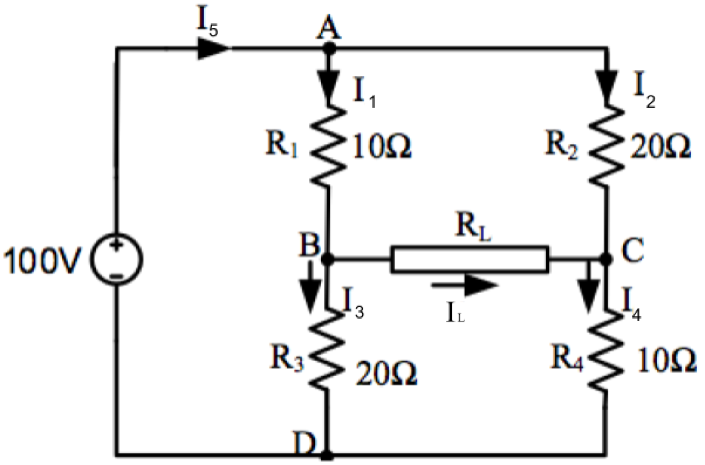a. For the circuit given in Figure 6 i. Find the load resistance RL that will receive maximum power and determine the maximum power. ii. Determine the power dissipated on the load when RL = 2 Ω and RL = 10 Ω. Figure 6 b. For the circuit shown in Figure 4, determine: iii. The power delivered to the load. iv. The impedance required in a matching network that will receive maximum power. Figure 4 1. R 1012 R2 32012...

• ### For the circuit shown below calculate Vout between points A and B when a load resistor...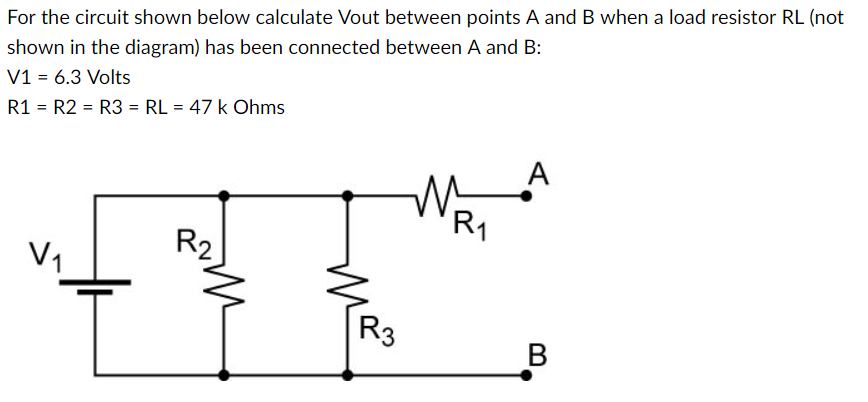For the circuit shown below calculate Vout between points A and B when a load resistor RL (not shown in the diagram) has been connected between A and B: V1-6.3 Volts R1 R2R3 RL 47k Ohms R2

• ### MULTISIM 10.65 The variable load resistor R¡ in the circuit shown in PSPICE Fig. P10.65 is...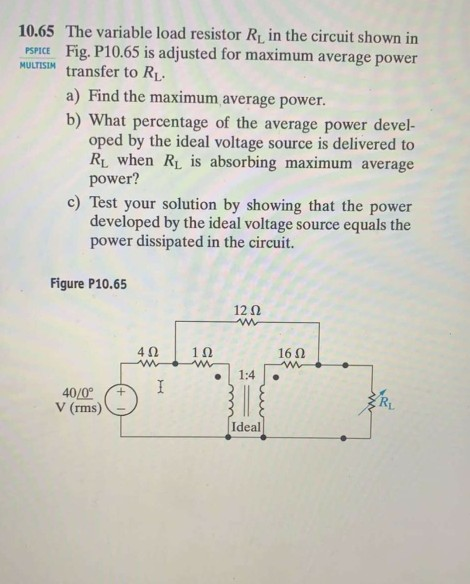MULTISIM 10.65 The variable load resistor R¡ in the circuit shown in PSPICE Fig. P10.65 is adjusted for maximum average power transfer to RL a) Find the maximum average power. b) What percentage of the average power devel- oped by the ideal voltage source is delivered to Rź when R is absorbing maximum average power? c) Test your solution by showing that the power developed by the ideal voltage source equals the power dissipated in the circuit. Figure P10.65 1212...

• ### In the voltage-divider circuit shown in the figure, the no-load value of vo is 5 V...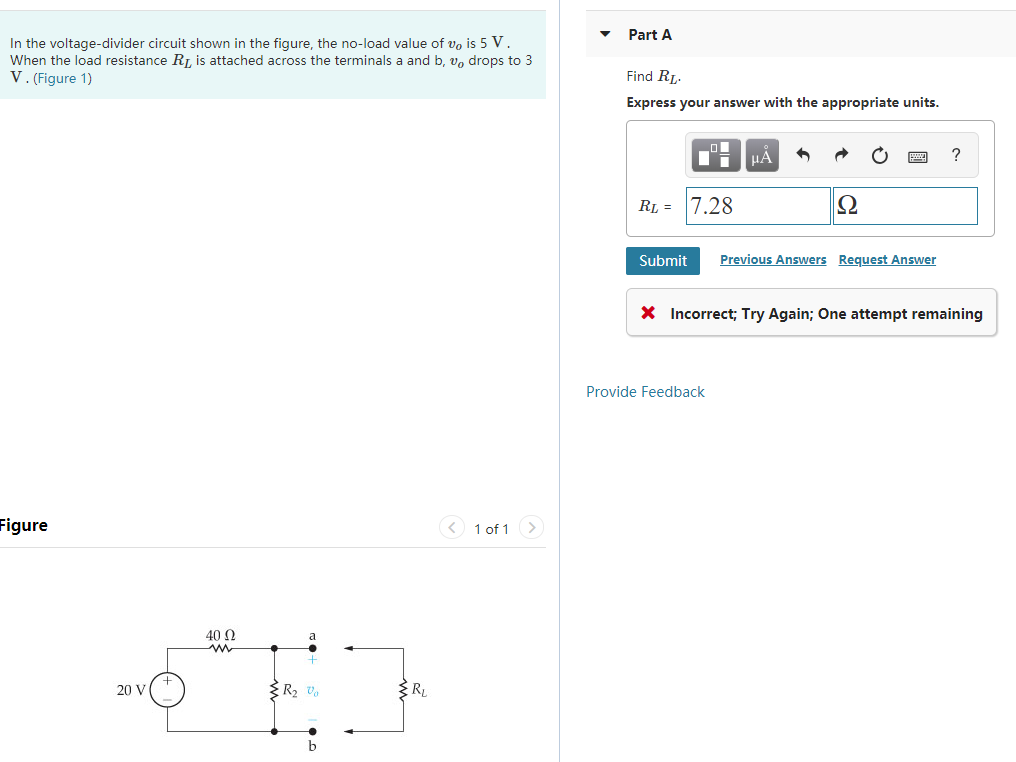In the voltage-divider circuit shown in the figure, the no-load value of vo is 5 V When the load resistance RI is attached across the terminals a and b, vo drops to 3 V.(Figure 1) PartA Find RL Express your answer with the appropriate units RI-7.28 Submit Previous Answers Request Answer Incorrect, Try Again: One attempt remaining Provide Feedback igure 1 of 1 40Ω 20 V R2 vo

• ### The circuit below uses an ideal opamp. Find the value of RL such that Vo is...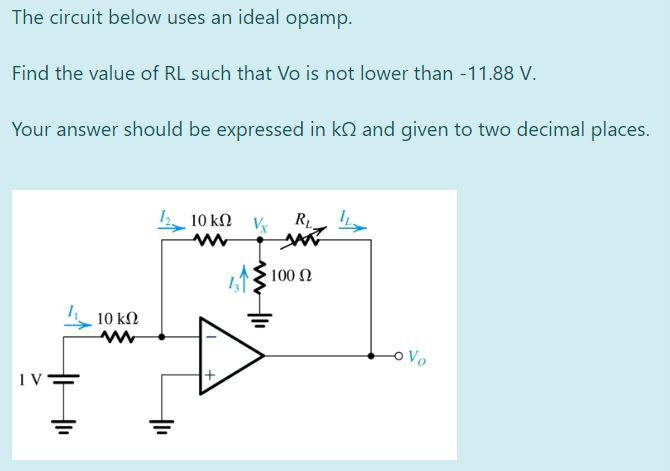The circuit below uses an ideal opamp. Find the value of RL such that Vo is not lower than -11.88 V Your answer should be expressed in kΩ and given to two decimal places. R, IL 100Ω 10 k2

• ### 0 40 V The no-load voltage across R2 in the voltage-divider circuit shown is shown is...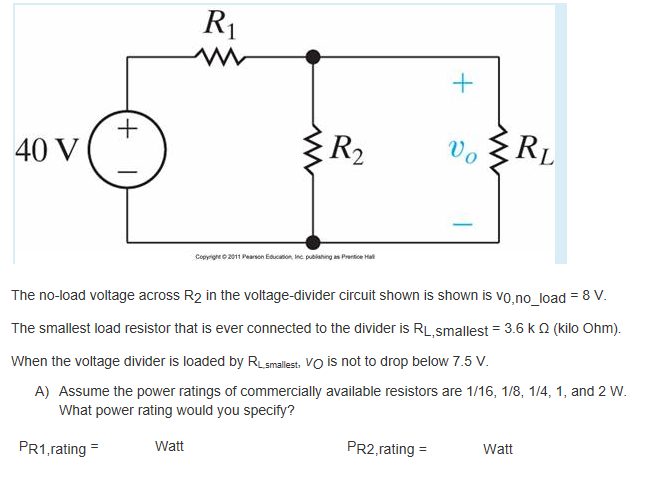0 40 V The no-load voltage across R2 in the voltage-divider circuit shown is shown is vo.no_load 8 V The smallest load resistor that is ever connected to the divider is RL,smallest 3.6 k Ω (kilo Ohm) When the voltage divider is loaded by RLsmallest, VO is not to drop below 7.5 V A) Assume the power ratings of commercially available resistors are 1/16, 1/8, 1/4, 1, and 2 W What power rating would you specify? PR1,rating Watt PR2.rating =...

• ### For the given circuit, find the following: The total load resistance of the circuit between circuit...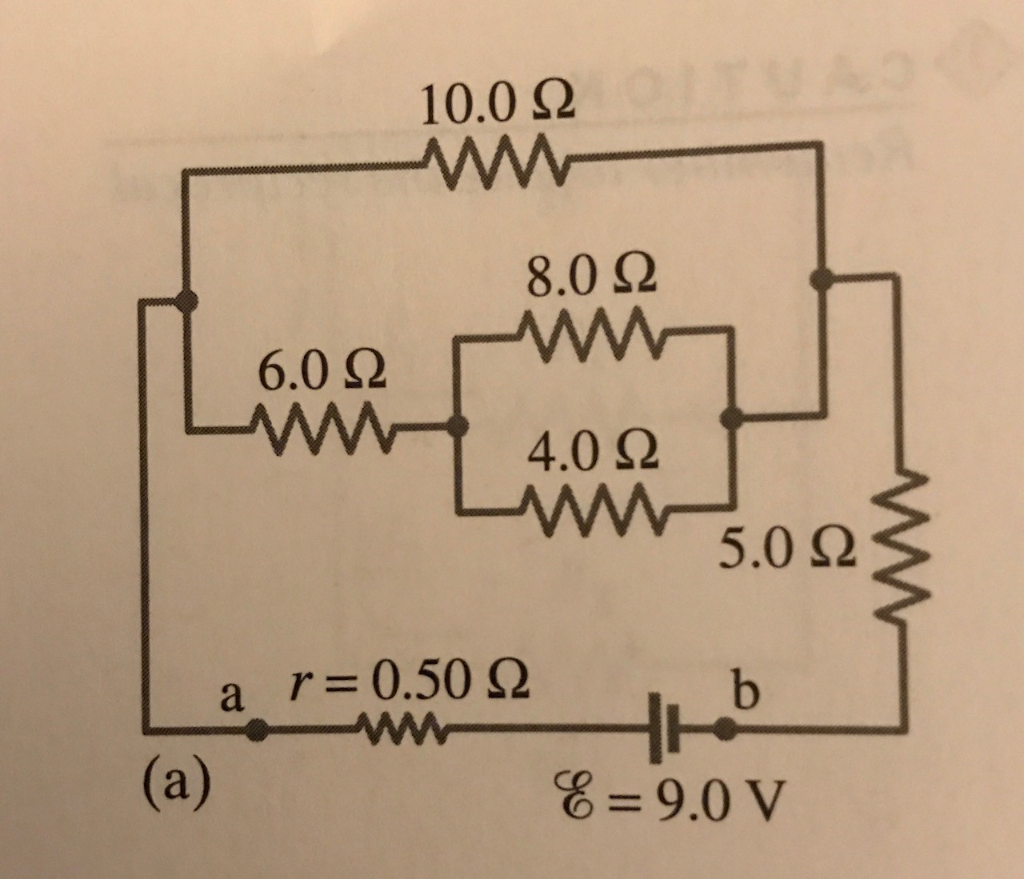For the given circuit, find the following: The total load resistance of the circuit between circuit points a and b. The current through each resistor. The voltage across each resistor. The power dissipated by each resistor. The power supplied by the battery. The terminal voltage, Vab. Please be as detailed as possible and explain each step. 10.0 Ω - 8.0 Ω 6.0 Ω 4.0 Ω LW 5.0 Ω a r=0.50 Ω - L (a) & = 9.0V

• ### Calculate the voltage at points A, B, and C, in the following circuit. Find the power...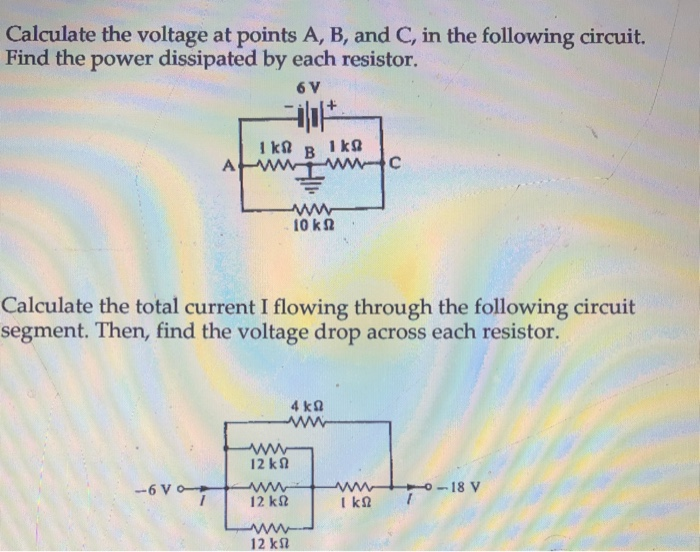Calculate the voltage at points A, B, and C, in the following circuit. Find the power dissipated by each resistor. 6 V Calculate the total current I flowing through the following circuit segment. Then, find the voltage drop across each resistor. -18 V 12 kΩ 12 k

• ### 7.2Ω 6Ω 2) For the circuit shown, find (a) the voltage Vo; (b) the power delivered...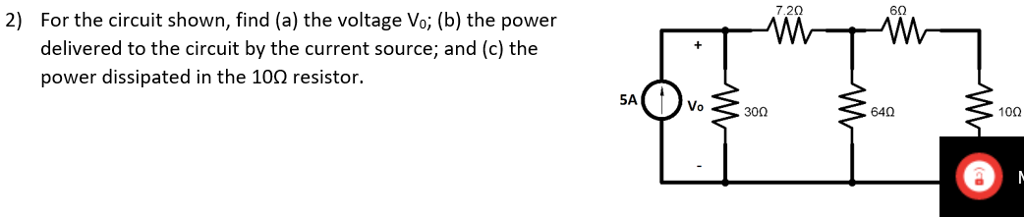7.2Ω 6Ω 2) For the circuit shown, find (a) the voltage Vo; (b) the power delivered to the circuit by the current source; and (c) the power dissipated in the 10Ω resistor. Vo 300 64Ω 10Ω

• ### 7 2Ω 6Ω For the circuit shown, find (a) the voltage Vo, (b) the power delivered...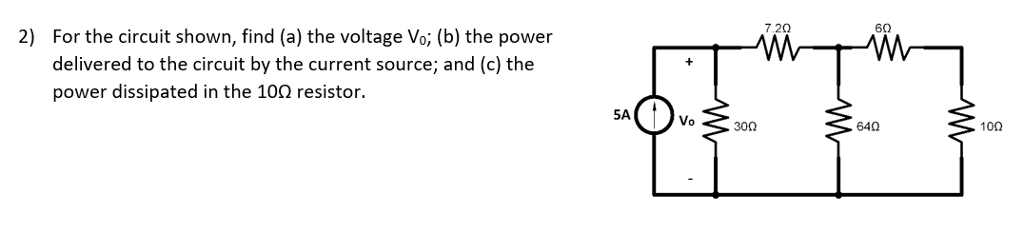7 2Ω 6Ω For the circuit shown, find (a) the voltage Vo, (b) the power delivered to the circuit by the current source; and (c) the power dissipated in the 10Ω resistor. 2) 5A Vo 30n 64Ω

Free Homework App